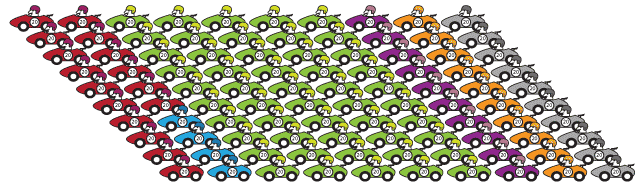### Connecting fractions and percentages

Percentages are another way of expressing fractions with denominators that are 10, 100, 1000 and so on.

Per centum comes from the Latin meaning 'out of one hundred'. So forty-five per cent or 45% means 45 out of one hundred and we write it as a fraction $$\dfrac{45}{100}.$$
If we tallied the colours of 100 cars that passed a point on the highway and 16 were red, we could say that 16 out of one hundred or $$\dfrac{16}{100} \text{or}\ 16\%$$ of those cars were red.Detailed description

We might show this by shading 16 out of 100 boxes on a grid.

There were 50 out of one hundred cars that were green. Another way of saying this is that fifty hundredths were green. This is the same as the fraction $$\dfrac{50}{100}$$ and the percentage is written as 50%.
We can show 50% on a 100-grid by colouring 50 of the 100 squares.

For a percentage such as 145%, when we write that as a fraction $$\dfrac{145}{100},$$ we notice that it is an improper fraction and can be written as a mixed number as $$1\dfrac{45}{100}\ \text{or}\ 1\dfrac{9}{20}.$$

We have shaded 100 hundredths in the first box. $$\dfrac{100}{100}$$ = 1 or 100%. We have shaded 45 hundredths in the second box. This is $$\dfrac{45}{100}$$ or 45%.